+300
3 to 1Aaron Judge
16.5% implied probability

+480
4.8 to 1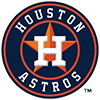Yordan Alvarez
11.3% implied probability

+850
8.5 to 1Mike Trout
6.9% implied probability

+950
9.5 to 1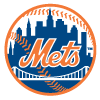Pete Alonso
6.3% implied probability

+1200
12 to 1C.J. Cron
5.1% implied probability

+1400
14 to 1Vladimir Guerrero
4.4% implied probability

+1600
16 to 1Giancarlo Stanton
3.9% implied probability

+1700
17 to 1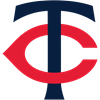Byron Buxton
3.7% implied probability

+2500
25 to 1Jose Ramirez
2.5% implied probability

+3000
30 to 1Bryce Harper
2.1% implied probability

+3000
30 to 1Shohei Ohtani
2.1% implied probability

+3500
35 to 1Kyle Schwarber
1.8% implied probability

+4000
40 to 1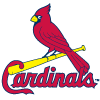Nolan Arenado
1.6% implied probability

+4800
48 to 1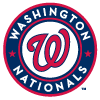Juan Soto
1.3% implied probability

+5000
50 to 1Anthony Rizzo
1.3% implied probability

+5500
55 to 1Hunter Renfroe
1.2% implied probability

+5500
55 to 1Rafael Devers
1.2% implied probability

+6000
60 to 1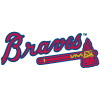Matt Olson
1.1% implied probability

+7000
70 to 1Austin Riley
0.9% implied probability

+7000
70 to 1George Springer
0.9% implied probability

+7000
70 to 1Manny Machado
0.9% implied probability

+7000
70 to 1Ronald Acuna
0.9% implied probability

+7000
70 to 1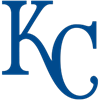Salvador Perez
0.9% implied probability

+8500
85 to 1Taylor Ward
0.8% implied probability

+8500
85 to 1Rowdy Tellez
0.8% implied probability

+8500
85 to 1Christian Walker
0.8% implied probability

+8500
85 to 1Rhys Hoskins
0.8% implied probability

+10000
100 to 1Joc Pederson
0.7% implied probability

+10000
100 to 1Willy Adames
0.7% implied probability

No changes have been recorded yet.
+10000
100 to 1Jose Altuve
0.7% implied probability

No changes have been recorded yet.
+10000
100 to 1Nick Castellanos
0.7% implied probability

+10000
100 to 1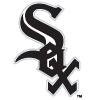Jose Abreu
0.7% implied probability

+10000
100 to 1Jorge Soler
0.7% implied probability

+10000
100 to 1Joey Gallo
0.7% implied probability

+11000
110 to 1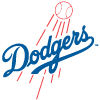Mookie Betts
0.6% implied probability

+11000
110 to 1Jazz Chisholm
0.6% implied probability

+11000
110 to 1Kyle Tucker
0.6% implied probability

+13000
130 to 1Christian Yelich
0.5% implied probability

+13000
130 to 1Franmil Reyes
0.5% implied probability

+13000
130 to 1Ozzie Albies
0.5% implied probability

+13000
130 to 1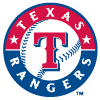Corey Seager
0.5% implied probability

+15000
150 to 1Jeremy Pena
0.4% implied probability

+15000
150 to 1Paul Goldschmidt
0.4% implied probability

+15000
150 to 1Jared Walsh
0.4% implied probability

+15000
150 to 1Matt Chapman
0.4% implied probability

+15000
150 to 1Luis Robert
0.4% implied probability

+20000
200 to 1Alex Bregman
0.3% implied probability

+20000
200 to 1Nelson Cruz
0.3% implied probability

+20000
200 to 1Marcell Ozuna
0.3% implied probability

+20000
200 to 1Francisco Lindor
0.3% implied probability

+20000
200 to 1Josh Bell
0.3% implied probability

+20000
200 to 1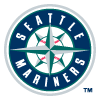Ty France
0.3% implied probability

+20000
200 to 1Max Muncy
0.3% implied probability

+20000
200 to 1Luke Voit
0.3% implied probability

+20000
200 to 1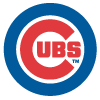Seiya Suzuki
0.3% implied probability

+25000
250 to 1Trevor Story
0.3% implied probability

+25000
250 to 1Tyler O'Neill
0.3% implied probability

+25000
250 to 1Bo Bichette
0.3% implied probability

+25000
250 to 1Kris Bryant
0.3% implied probability

+25000
250 to 1Freddie Freeman
0.3% implied probability

+25000
250 to 1Javier Baez
0.3% implied probability

+25000
250 to 1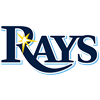Wander Franco
0.3% implied probability

+25000
250 to 1Randal Grichuk
0.3% implied probability

+25000
250 to 1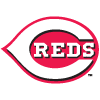Joey Votto
0.3% implied probability

+25000
250 to 1Marcus Semien
0.3% implied probability

+25000
250 to 1Adam Duvall
0.3% implied probability

+25000
250 to 1Julio Rodriguez
0.3% implied probability

+25000
250 to 1Brandon Lowe
0.3% implied probability

+25000
250 to 1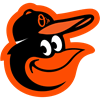Ryan Mountcastle
0.3% implied probability

+25000
250 to 1Teoscar Hernandez
0.3% implied probability

+50000
500 to 1Carlos Correa
0.1% implied probability

+50000
500 to 1Bobby Witt
0.1% implied probability

+50000
500 to 1Mitch Haniger
0.1% implied probability

+50000
500 to 1Austin Meadows
0.1% implied probability

+50000
500 to 1J.D. Martinez
0.1% implied probability